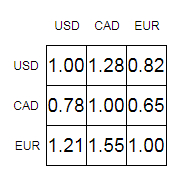# Program to Find Out Currency Arbitrage in Python

Suppose we have one N x N table of currency exchange rates. We have to check whether there is some sequence of trades we can make or not. Now starting with some amount A of any currency, we can end up with some amount greater than A of that currency. There are no transaction costs and we can also trade fractional quantities.The value at entry [I, j] in this matrix represents the amount of currency j we can buy with one unit of currency i. Now consider currency 0 is USD, 1 is CAD and 2 is EUR. We can make an arbitrage with the following −

• Sell 1 CAD for 0.65 EUR

• Sell 0.65 EUR for 0.7865 USD (0.65 * 1.21)

• Sell 0.7865 USD for 1.00672 CAD (0.65 * 1.21 * 1.28)

So, if the input is like

 1 1.28 0.82 0.78 1 0.65 1.21 1.55 1

then the output will be True.

To solve this, we will follow these steps −

• for i in range 0 to size of matrix, do

• for j in range 0 to size of matrix, do

• matrix[i,j] := −log base 2 value of (matrix[I, j])

• v := row count of matrix

• for k in range 0 to v, do

• for i in range 0 to v, do

• for j in range 0 to v, do

• matrix[I, j] := minimum of matrix[I, j] and (matrix[I, k] + matrix[k, j])

• return True if any of the items in the diagonal of the matrix is non−zero.

Let us see the following implementation to get better understanding −

## Python

Live Demo

import math
class Solution:
def solve(self, matrix):
for i in range(len(matrix)):
for j in range(len(matrix)):
matrix[i][j] = −math.log(matrix[i][j], 2)
v = len(matrix)
for k in range(0, v):
for i in range(0, v):
for j in range(0, v):
matrix[i][j] = min(matrix[i][j], matrix[i][k] + matrix[k][j])
return any(matrix[i][i] < 0 for i in range(len(matrix)))
ob = Solution()
matrix = [
[1, 1.28, 0.82],
[0.78, 1, 0.65],
[1.21, 1.55, 1]
]
print(ob.solve(matrix))

## Input

matrix = [
[1, 1.28, 0.82],
[0.78, 1, 0.65],
[1.21, 1.55, 1]
]

## Output

True

Updated on: 15-Dec-2020

840 Views